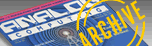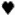`A.N.A.L.O.G. ISSUE 79 / DECEMBER 1989 / PAGE 6`

 READER COMMENT Convertor Problems ĀĀ Ā The Ultimate Graphics File Convertor (ANALOG Computing, May '89) listing was printed incorrectly. When I unscrambled the listing and typed it in, the program got confused when trying to load Newsroom files and also when going from Graphics 8 to Newsroom. Please talk to Dr. Brilliant and have this program corrected, since it seems to be a very useful utility. -Dave Faxon Kingston, NH ...The Doc Replies ĀĀ ĀYes, indeed, there are problems with UGFC. The problem arose because I had a disk failure just before I finished the program. I thought I had reconstructed the program, but in fact I had used an earlier version, which was written to be used only with a specific Newsroom picture file. The lines below correct the problems. Load the original UGFC program and delete lines 5050 to 5130 and line 6015. Then enter the lines below and resave the program. -Lee Brilliant, M.D. Granada Hills, CA 631 IF T0=4 AND COLR-COLL>247 THEN COL R=COLR-1 632 RETURN 681 IF T0=4 AND COLR-COLL>247 THEN COL L=COLL+1 682 RETURN 5050 COUNT=(N-1)/32:GOSUB 100:GOSUB 13 020:? #6;CHR\$(125) 5060 DIR\$(1)="":DIRS(7680)="":DIR\$(2 )=DIR\$:GOSUB 10000 5970 GOSUB 180:IF X=255 THEN 5200 5080 LO=X:GOSUB 180:HI=X:GOSUS 180:OFF =X 5090 SECT2=SECT:BYTE2=BYTE:SECT=LO+256 *HI:BYTE=OFF+1:IF BYTE>128 THEN BYTE=1 :SECT=SECT+1 5100 GOSUB 10000:GOSUB 180:ROWT=X:GOSU B 180:ROWB=X;GOSUB 180:COLL=X:GOSUB 18 0:COLR=X 5110 C=INT((COLR-COLL)/8)+1:R=ROWB-ROW T+1:AD=ADR(DIR\$):SIZE=C*R:COUNT=0:RCOU NT=0:OFF=40*(ROWT-1)+INT(COLL/8) 5115 OFF1=OFF 5120 START=AD+OFF:TERMINATE=0:GOSUB 30 0:SHIFT=COLL-INT(COLL/8)*8:IF SHIFT=0 THEN 5125 5122 FOR N=1 TO 5HIFT:A=USR(ADR(ROR\$), ADR(DIR\$),7680):NEXT N 5125 A=USR(ADR(ORA\$),ADR(DIR\$),PEEK(88 )+256*PEEK(89),7680) 5130 SECT=SECT2:BYTE=BYTE2:GOTO 5060 5200 IF BUF\$(BYTE,BYTE)<>CHR\$(255) THE N 5080 9005 A=USR(ADR(MOVE\$),ADR(CURSOR\$),153 6,LEN(CURSORS)):A=USR(1560,0,8,191,247 ) 9010 D=4:FLAG=0:COLL=8:ROWT=0:COLR=247 :ROWB=191:POKE 764,33:GOSUB 500 9142 D=COLL-8:IF D<0 THEN COLR=COLR-D: COLL=8 ALL LETTERS TO BE CONSIDERED FOR PUBLICATION SHOULD BE ADDRESSED TO:  ANALOG, READER COMMENT P.O. BOX 1413-M.O. MANCHESTER, CT 06040-1413